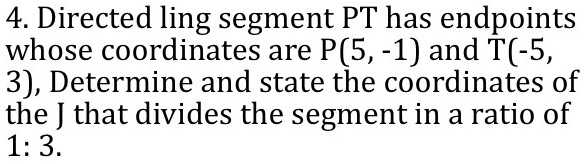### ¿Todavía tienes preguntas de matemáticas?

Pregunte a nuestros tutores expertos
Algebra
Pregunta4. Directed ling segment PT has endpoints whose coordinates are P(5, $$- 1 )$$ and T( $$- 5$$ , 3), Determine and state the coordinates of the J that divides the segment in a ratio of $$1 : 3$$ .

the slope=-$$\frac{2}{5}$$